#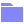Common Library (PQL)

• Family: PQL
• ## Function Categories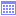Date-Time These functions are used to perform calculations on dates and times.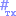Formats These functions represent some predefined formulas for formatting numbers and dates.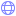Geo These functions are used to perform geospatial distance calculations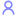Identity These functions are used to perform derive details of the logged in user's identity and rights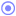Logical These functions are used for performing logical and conditional operations.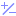Math These functions are used for performing mathematical functions and operations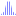Statistical These functions are used for performing statistical and aggregate functions and operations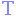String These functions are used for manipulating text strings.

## Overview

Common functions are a set of methods that are found in most programmatic and mathematical function libraries. These functions are used to perform logic on basic data without concern for data structures, context, or even source. As such they can run almost anywhere and are common to all parts of the calculation engine.

The Common library includes numerous methods for handling math, dates, strings and logical flows. It also includes a collection of functions that will help developers identify Pyramid users (and their details) plus methods for strengthening the calculation power of the other parts of the calculation engine.

Common calculations are usually done with standard libraries found in Java, C#, VB, VBA and C++.